### 6.11. Perspective

#### 6.11.1. Overview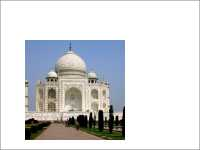Original image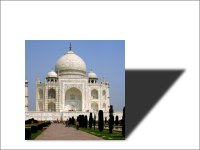Perspective applied

This filter adds a perspective shadow to the selected region or alpha-channel as a layer below the active layer. You may select color, length and direction of the shadow as well as the distance of the horizon.

If necessary, the filter may resize the image. But it will not add a background to make the shadow visible.

#### 6.11.2. Activate the filter

You can access this filter in the image window menu through FiltersLight and ShadowPerspective….

#### 6.11.3. Options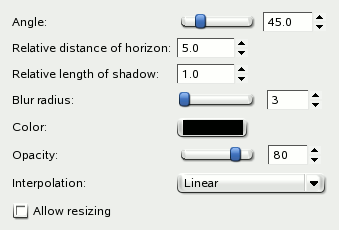Angle

The angle determines the direction of the shadow or the imaginary source of light, respectively. Values range from 0° to 180°, where 90° represents a light source just in front of the selection or layer. For angles less than 90°, the shadow is at the right side, so the light source is on the left. For angles greater than 90°, it's the other way round. Tip: think of the slider's handle as source of light.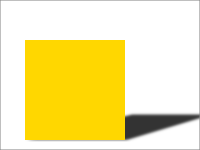Angle 15°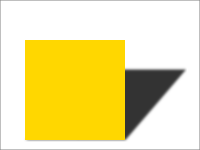Angle 45° (default)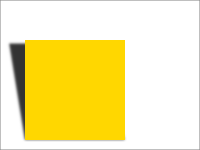Angle 105° (90° + 15°)

Relative distance of horizon

This option determines how far away the imaginary horizon is. The relative distance is the distance from the ground-line of the selection or layer, the unit of measurement is the height of the selection or layer.

Value range is from 0.1 to 24.1, where 24.1 means (nearly) infinite. Note that the relative length of shadow must not exceed the distance of horizon.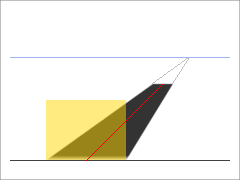Angle = 45°. Distance = 2.4. Length = 1.8.

In the example above, the yellow area is the selection the filter is applied to. The blue line at the top represents the imaginary horizon. The angle between the selection's ground-line and the red line is 45°. The length of the red line is 1.8 times the height of the yellow selection. Extended to the horizon, the length is 2.4 times the selection's height.

Relative length of shadow

With this option you can set the length of shadow with respect to the height of the selection or layer. In the above example, the red line represents the length of shadow, its length is 1.8 relative to the height of the yellow selection.

Value range is from 0.1 to 24.1, although the length of shadow must not exceed the relative distance of horizon - you can't go beyond the horizon.Length = 1.0 (default)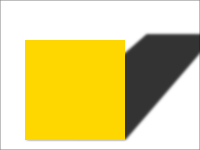Length = 1.5

After creating the shadow, a Gaussian blur with the specified radius is applied to the shadow layer, resulting in the realistic appearance of the shadow.Blur radius = 3 (default)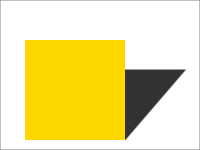Without blur (blur radius = 0)

Color

Of course, the default color of the shadow is black. But a click on the button opens the color selector, where you may select any other color.

Opacity

The shadow's opacity is the opacity of the new layer containing the shadow (see 第 1.1 节 “Layer Properties”). It defaults to 80%, but you may select any other value from 0 (full transparency) to 100 (full opacity) here. After applying the filter to an image you can change the opacity in the layers dialog.

Interpolation

This drop-down list lets you choose the method of interpolation used when the shadow layer is transformed, for example rotated by the specified angle. Using None will usually result in aliasing, using any interpolation method may change the color of the shadow in some areas. Linear is a good choice.

Allow resizing

If enabled, the filter will resize the image if that is needed to make place for the shadow.

In the example below, the yellow area is the active selection, background is light blue. The white area has been added after resizing to make the shadow visible.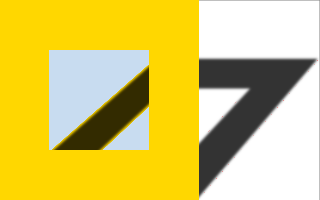Allow resizing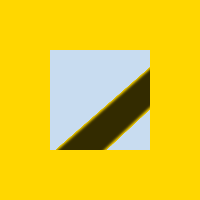Don't allow resizing